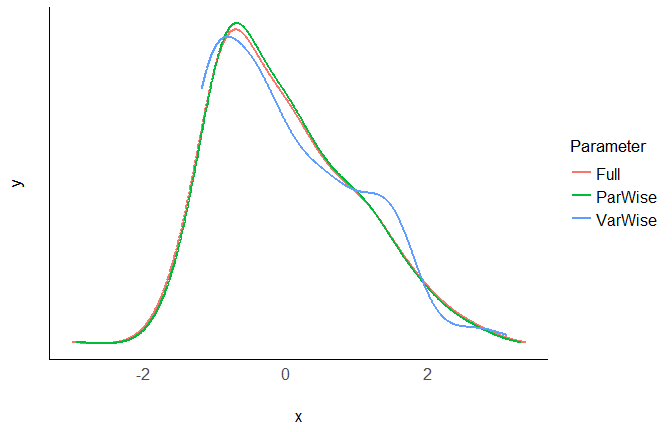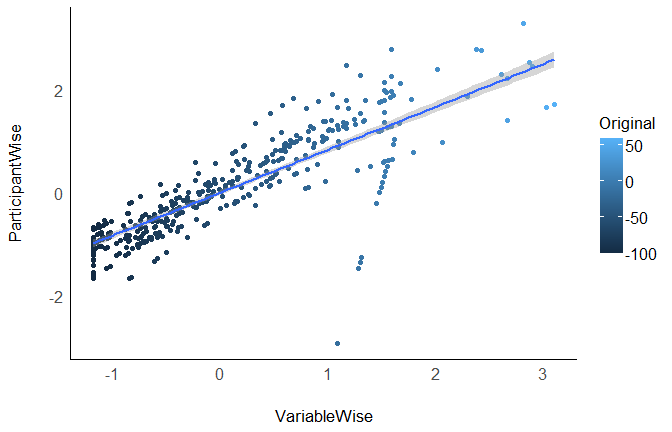# Introduction

To make sense of their data and effects, scientists might want to standardize (Z-score) their variables. They become unitless, expressed only in terms of deviation from an index of centrality (e.g., the mean or the median). However, aside from some benefits, standardization also comes with challenges and issues, that the scientist should be aware of.

## Normal vs. Robust

The `effectsize` package offers two methods of standardization.

[TO DO]

## Variable-wise vs. Participant-wise

Standardization is an important step and extra caution is required in repeated-measures designs, in which there are three ways of standardizing data:

• Variable-wise: The most common method. A simple scaling of each column.
• Participant-wise: Variables are standardized “within” each participant, i.e., for each participant, by the participant’s mean and SD.
• Full: Participant-wise first and then re-standardizing variable-wise.

Unfortunately, the method used is often not explicitly stated. This is an issue as these methods can generate important discrepancies (that can in turn contribute to the reproducibility crisis). Let’s investigate these 3 methods.

# The data

We will take the `emotion` dataset in which participants were exposed to negative pictures and had to rate their emotions (valence) and the amount of memories associated with the picture (autobiographical link). One could make the hypothesis that for young participants with no context of war or violence, the most negative pictures (mutilations) are less related to memories than less negative pictures (involving for example car crashes or sick people). In other words, we expect a positive relationship between valence (with high values corresponding to less negativity) and autobiographical link.

Let’s have a look at the data, averaged by participants:

``````library(dplyr)

# Discard neutral pictures (keep only negative)
df <- emotion %>%
filter(Emotion_Condition == "Negative")

# Summary
df %>%
group_by(Participant_ID) %>%
summarise(n_Trials = n(),
Valence_Mean = mean(Subjective_Valence, na.rm=TRUE),
Valence_SD = sd(Subjective_Valence, na.rm=TRUE),

As we can see from the means and SDs, there is a lot of variability between and within participants (between their means and their individual SD).

# Standardize

We will create three dataframes standardized with each of the three techniques.

``````library(effectsize)

Z_VariableWise <- df %>%
standardize()

Z_ParticipantWise <- df %>%
group_by(Participant_ID) %>%
standardize()

Z_Full <- df %>%
group_by(Participant_ID) %>%
standardize() %>%
ungroup() %>%
standardize() ``````

# Effect of Standardization

Let’s see how these three standardization techniques affected the Valence variable.

## At a general level

``````# Create a convenient function to print
print_summary <- function(data){
paste0(
paste0(deparse(substitute(data)), ": "),
paste(round(mean(data[["Subjective_Valence"]]), 3),
"+-",
round(sd(data[["Subjective_Valence"]]), 3)),
paste0(" [",
round(min(data[["Subjective_Valence"]]), 3),
",",
round(max(data[["Subjective_Valence"]]), 3),
"]")
)
}

# Check the results
print_summary(Z_VariableWise)``````
`````` "Z_VariableWise: 0 +- 1 [-1.178,3.101]"
``````
``print_summary(Z_ParticipantWise)``
`````` "Z_ParticipantWise: 0 +- 0.98 [-2.933,3.293]"
``````
``print_summary(Z_Full)``
`````` "Z_Full: 0 +- 1 [-2.992,3.36]"
``````

The means and the SD appear as fairly similar (0 and 1)…

## Distribution

``````library(bayestestR)
library(see)

data.frame(VarWise = Z_VariableWise\$Subjective_Valence,
ParWise = Z_ParticipantWise\$Subjective_Valence,
Full = Z_Full\$Subjective_Valence) %>%
estimate_density(method="kernSmooth") %>%
plot() +
see::theme_modern()``````and the distributions appear to be similar…

## At a participant level

``````# Create convenient function
print_participants <- function(data){
data %>%
group_by(Participant_ID) %>%
summarise(Mean = mean(Subjective_Valence),
SD = sd(Subjective_Valence)) %>%
mutate_if(is.numeric, round, 2) %>%

}

# Check the results
print_participants(Z_VariableWise)``````
``````# A tibble: 5 x 3
Participant_ID  Mean    SD
<fct>          <dbl> <dbl>
1 10S            -0.05  1.15
2 11S            -0.46  1
3 12S            -0.03  0.72
4 13S            -0.19  0.64
5 14S            -0.01  0.71
``````
``print_participants(Z_ParticipantWise)``
``````# A tibble: 5 x 3
Participant_ID  Mean    SD
<fct>          <dbl> <dbl>
1 10S                0     1
2 11S                0     1
3 12S                0     1
4 13S                0     1
5 14S                0     1
``````
``print_participants(Z_Full)``
``````# A tibble: 5 x 3
Participant_ID  Mean    SD
<fct>          <dbl> <dbl>
1 10S                0  1.02
2 11S                0  1.02
3 12S                0  1.02
4 13S                0  1.02
5 14S                0  1.02
``````

Ok so there are some differences here….

# Correlation

Let’s do a correlation between the variable-wise and participant-wise methods.

``````library(ggplot2)

cor.test(Z_VariableWise\$Subjective_Valence, Z_ParticipantWise\$Subjective_Valence)``````
``````
Pearson's product-moment correlation

data:  Z_VariableWise\$Subjective_Valence and Z_ParticipantWise\$Subjective_Valence
t = 35, df = 454, p-value <2e-16
alternative hypothesis: true correlation is not equal to 0
95 percent confidence interval:
0.82 0.87
sample estimates:
cor
0.85
``````
``````data.frame(Original = df\$Subjective_Valence,
VariableWise = Z_VariableWise\$Subjective_Valence,
ParticipantWise = Z_ParticipantWise\$Subjective_Valence) %>%
ggplot(aes(x=VariableWise, y=ParticipantWise, colour=Original)) +
geom_point() +
geom_smooth(method="lm") +
see::theme_modern()``````While the three standardization methods roughly present the same characteristics at a general level (mean 0 and SD 1) and a similar distribution, their values are not exactly the same!

# Test

Let’s now answer to the original question by investigating the linear relationship between valence and autobiographical link. We can do this by running a mixed model with participants entered as random effects.

``````library(lme4)
library(parameters)

# Convenient function
print_model <- function(data){
type_name <- deparse(substitute(data))
lmer(Subjective_Valence ~ Autobiographical_Link + (1|Participant_ID), data=data) %>%
parameters() %>%
mutate(Type = type_name,
Coefficient = round(Coefficient, 3),
p = round(p, 3)) %>%
select(Type, Coefficient, p)
}

# Run the model on all datasets
rbind(print_model(df),
print_model(Z_VariableWise),
print_model(Z_ParticipantWise),
print_model(Z_Full))``````
``````Type              | Coefficient |     p
---------------------------------------
df                |        0.09 | 0.173
Z_VariableWise    |        0.07 | 0.173
Z_ParticipantWise |        0.08 | 0.080
Z_Full            |        0.08 | 0.080
``````

As we can see, variable-wise standardization only affects the coefficient (which is expected, as it changes the unit), but not the test statistics (the p-value, in this case). However, using participant-wise standardization does affect the coefficient and the p-value. No method is better or more justified, and its choice depends on the specific case, context, data and goal.

# Conclusion

1. Standardization can be useful in some cases and should be justified
2. Variable and Participant-wise standardization methods produce “in appearance” similar data
3. Variable and Participant-wise standardization can lead to different results
4. The choice of the method can strongly influence the results and thus, should be explicitly stated

We showed here yet another way of sneakily tweaking the data that can change the results. To prevent its use for bad practices (e.g., p-hacking), we can only support the generalization of open-data, open-analysis and preregistration**